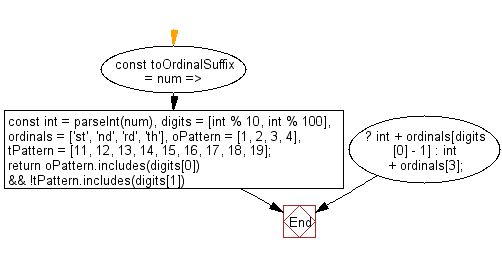# JavaScript: Add an ordinal suffix to a number

## JavaScript fundamental (ES6 Syntax): Exercise-122 with Solution

Write a JavaScript program to add an ordinal suffix to a number.

• Use the modulo operator (%) to find values of single and tens digits.
• Find which ordinal pattern digits match.
• If digit is found in teens pattern, use teens ordinal.

Sample Solution:

JavaScript Code:

``````//#Source https://bit.ly/2neWfJ2
const toOrdinalSuffix = num => {
const int = parseInt(num),
digits = [int % 10, int % 100],
ordinals = ['st', 'nd', 'rd', 'th'],
oPattern = [1, 2, 3, 4],
tPattern = [11, 12, 13, 14, 15, 16, 17, 18, 19];
return oPattern.includes(digits) && !tPattern.includes(digits)
? int + ordinals[digits - 1]
: int + ordinals;
};

console.log(toOrdinalSuffix('1'));
console.log(toOrdinalSuffix('4'));
console.log(toOrdinalSuffix('50'));
console.log(toOrdinalSuffix('123'));
```
```

Sample Output:

```1st
4th
50th
123rd
```

Flowchart:Live Demo:

See the Pen javascript-basic-exercise-122-1 by w3resource (@w3resource) on CodePen.

Improve this sample solution and post your code through Disqus

What is the difficulty level of this exercise?

Test your Programming skills with w3resource's quiz.

﻿